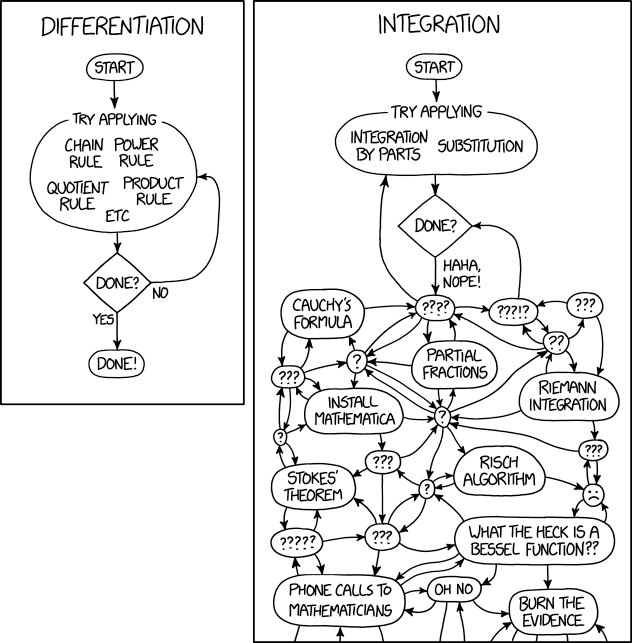# Differentiation and Integration

As I tell my calculus students, differentiation is a science. There are rules to follow, but if you follow them carefully, you can compute the derivative of anything. This leads to one of my favorite classroom activities. However, integration is as much art as science; for example, see my series on different techniques for computing$\displaystyle \int_0^{2\pi} \frac{dx}{\cos^2 x + 2 a \sin x \cos x + (a^2 + b^2) \sin^2 x}$

The contrast between differentiation and integration was more vividly illustrated in a recent xkcd webcomic:Source: https://xkcd.com/2117/